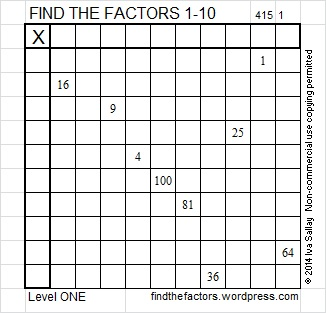# 89 and Level 1

• 89 is a prime number.
• Prime factorization: 89 is prime.
• The exponent of prime number 89 is 1. Adding 1 to that exponent we get (1 + 1) = 2. Therefore 89 has exactly 2 factors.
• Factors of 89: 1, 89
• Factor pairs: 89 = 1 x 89
• 89 has no square factors that allow its square root to be simplified. √89 ≈ 9.43398How do we know that 89 is a prime number? If 89 were not a prime number, then it would be divisible by at least one prime number less than or equal to √89 ≈ 9.4. Since 89 cannot be divided evenly by 2, 3, 5, or 7, we know that 89 is a prime number.

89 is never a clue in the FIND THE FACTORS puzzles.This week’s puzzles and last week’s solutions: 10 Factors 2014-04-14This site uses Akismet to reduce spam. Learn how your comment data is processed.